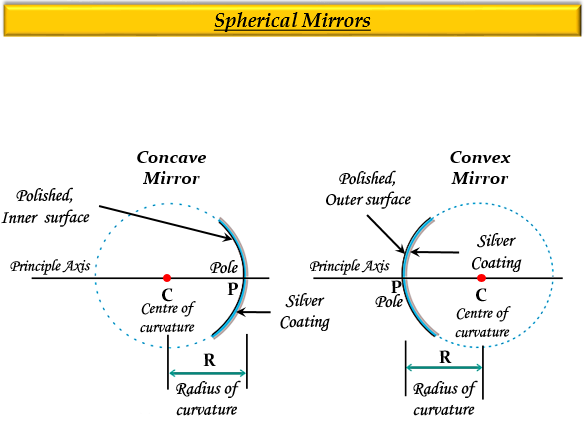# The shiny outer surface of a hollow sphere of aluminium of radius 50 cm is to be used as a mirror:(a) What will be the focal length of this mirror?(b) Which type of spherical mirror will it provide?(c) State whether this spherical mirror will perge or converge light rays.

(a) Given:

The radius of curvature of the mirror, $R$ = 50 cm.

We know that the focal length, $f$ is equal to the half of the radius of curvature, $R$, it is given as:

$f=\frac {1}{2} \times {R}$

Now, putting the value $R$ in the above equation we get-

$f=\frac {1}{2} \times {50}$

$f=25cm$

Hence, the focal length of this mirror will be 25 cm.

(b) Since the outer surface is shiny, it will provide a convex mirror.

(c) This spherical mirror will diverge the light rays.

Explanation

If a spherical mirror, containing the inner surface shiny or polished, then it will be a concave mirror, whereas a spherical mirror, containing the outer surface shiny or polished, then it will be a convex mirror.

A concave mirror converges the light rays due to which it is also known as a converging mirror, whereas a convex mirror diverges the light rays, and also known as a diverging mirror.Updated on: 10-Oct-2022

2K+ Views